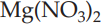×
Get Full Access to Introductory Chemistry - 5 Edition - Chapter 13 - Problem 76p
Get Full Access to Introductory Chemistry - 5 Edition - Chapter 13 - Problem 76p

×

# What volume of a 0.35 M Mg(NO3)2 solution contains 87 g of Mg(NO3)2?ISBN: 9780321910295 34

## Solution for problem 76P Chapter 13

Introductory Chemistry | 5th Edition

• Textbook Solutions
• 2901 Step-by-step solutions solved by professors and subject experts
• Get 24/7 help from StudySoup virtual teaching assistantsIntroductory Chemistry | 5th Edition

4 5 1 239 Reviews
20
4
Problem 76P

Problem 76P

What volume of a 0.35 M Mg(NO3)2 solution contains 87 g of Mg(NO3)2?

Step-by-Step Solution:

Problem 76P

What volume of a 0.35 Msolution contains 87 g of?

Step by Step Solution

Step 1 of 3

Number of moles can be calculated by the following equation;.....(1)

Step 2 of 3

Step 3 of 3

##### ISBN: 9780321910295

This textbook survival guide was created for the textbook: Introductory Chemistry, edition: 5. The answer to “What volume of a 0.35 M Mg(NO3)2 solution contains 87 g of Mg(NO3)2?” is broken down into a number of easy to follow steps, and 13 words. This full solution covers the following key subjects: contains, solution, Volume. This expansive textbook survival guide covers 19 chapters, and 2046 solutions. Introductory Chemistry was written by and is associated to the ISBN: 9780321910295. The full step-by-step solution to problem: 76P from chapter: 13 was answered by , our top Chemistry solution expert on 05/06/17, 06:45PM. Since the solution to 76P from 13 chapter was answered, more than 1181 students have viewed the full step-by-step answer.

Unlock Textbook Solution